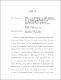## From Nanometers to Light Years: Exploration of the Early Universe with Gamma-ray Bursts and Development of Photonic Spectrographs for AstronomyRecent space- and ground-based studies of the circumgalactic medium (CGM) around nearby galaxies have revealed the dynamic interplay between the galaxy ecosystem and surrounding CGM using bright background quasars. In this thesis, we extend this investigation to higher redshifts by using the bright afterglows of gamma-ray bursts (GRBs) as background sources probing the CGM of their own host galaxies. We compiled a sample of 27 high-resolution (R $>$ 6000) rest-frame UV spectra of GRB afterglows in a redshift range (2 $\lesssim$ z $\lesssim$ 6); we call this the CGM-GRB sample'. We find stronger blue wings in high-ionization species (Si IV, C IV) compared to the low-ionization species (Si II, Fe II), indicative of the presence of ubiquitous warm outflows in the GRB hosts at high redshifts. Using kinematic models, we estimated typical values of CGM properties (for the sample) such as CGM mass (10$^{9.8}$ $\mathrm{M_{\odot}}$) and outflow launch velocity (300 km s$^{-1}$). Further, by comparing our results with previous C IV absorption studies, we find a possible CGM-galaxy co-evolution. Over the course of evolution of present-day galaxies with $\mathrm{M_{*}}$ $>$ $10^{10}$ $\mathrm{M_{\odot}}$, the ratio of C IV mass in the CGM to the stellar mass remains fairly uniform, such that log$\mathrm{(M_{C IV}/M_{*})} \sim -4.5$ within $\pm$0.5 dex from $z\sim4$ to $z\sim0$, suggesting CGM-galaxy co-evolution. Next, we embarked on a search for possible relations between the outflow properties and those of the host galaxies such as $\mathrm{M_*}$, star formation rate (SFR), and specific SFR (= SFR/$\mathrm{M_*}$). To estimate the total SFR, we first investigated the degree of dust obscuration in the massive GRB hosts in our sample by comparing radio- and UV-based star-formation rates. We inferred that the GRB hosts in our sample are not heavily dust obscured, and hence, their SFRs can be estimated reliably using the established dust-correction methods. For the outflow-galaxy correlations, we focused on three outflow properties $-$ outflow column density ($\mathrm{N_{out}}$), maximum outflow velocity ($\mathrm{V_{max}}$), and normalized maximum velocity ($\mathrm{V_{norm}}$ = $\mathrm{V_{max}/V_{circ, halo}}$, where $\mathrm{V_{circ, halo}}$ is the halo circular velocity). We observe clear trends of $\mathrm{N_{out}}$ and $\mathrm{V_{max}}$ with increasing SFR in high-ion-traced outflows. These correlations indicate that these high-ion outflows are driven by star formation at these redshifts (in the mass range $\mathrm{log(M_*/M_{\odot})}$ $\sim$ $9-11$). We also, for the first time, observe a strong ($>$ 3$\sigma$) trend of normalized velocity decreasing with halo mass and increasing with sSFR at high redshifts, suggesting that outflows from low-mass halos and high sSFR galaxies are most likely to escape and enrich the outer CGM and IGM with metals. Thus, we demonstrate GRB afterglows as a method to uncover CGM-galaxy co-evolution and outflow-galaxy correlations at high redshifts, which constitute an important piece of the galaxy growth puzzle and cosmic metal enrichment. Next, we set out to develop a new tool $-$ an on-chip photonic spectrograph $-$ which will eventually expand our investigation to the first galaxies in the universe ($z > 6$). Astrophotonics is the application of versatile photonic technologies to channel, manipulate, and disperse guided light to efficiently achieve various scientific objectives in astronomy in a miniaturized form factor. We used the concept of arrayed waveguide gratings (AWG) to develop an on-chip photonic spectrograph in the H band ($1.45-1.65$ $\mu m$) with a moderate resolving power of $\sim$1500, a peak throughput of $\sim$23\%, and a size of only 1.5 cm $\times$ 1.5 cm. Various practical aspects of implementing AWGs as astronomical spectrographs are also discussed, including a) the coupling of the light between the fibers and AWGs, b) cleaving at the output focal plane of the AWG to provide continuous wavelength coverage, and c) a multi-input AWG design to receive light from multiple single-mode fibers at a time and produce a combined spectrum. Finally, we built a cross-dispersion setup which will orthogonally separate the overlapping spectral orders in the AWG and thus image the full spectrum on the detector. The AWG will be incorporated with this setup in the near future to get the spectrograph ready for our first on-sky test. The work conducted in this thesis is a crucial stepping stone towards building a high-throughput, miniaturized spectrograph for the next generation of ground-, balloon-, and space-based telescopes. With the nano-scale fabrication on a chip, we are poised to unravel the mysteries of galaxies billions of light years away, making this thesis a truly \textit{Nanometers to Light Years'} journey.